Smartick is a fun way to learn math!May18

# The Relationship between Clocks and Angles

In today’s post, we’re going to take a look at the relationship between clocks and angles.

More specifically, we’ll see the kinds of angles that can be formed by the hands of a clock and how to calculate them.

On a clock, we can see different kinds of angles based on its amplitude; void, acute, straight, obtuse, flat angles—even a full angle.

Let’s check out some examples.

In this first example, we can see that the angle formed by the hands is an acute angle because it’s less than 90° and more than 0°.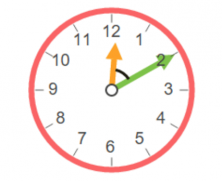On the next clock, where the hands point to 6 o’clock sharp, the hands form a 180° flat angle.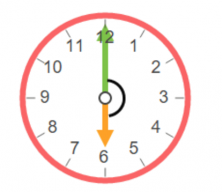In our last example, we have a clock where the hands form a full angle, that is, an angle that’s 360°.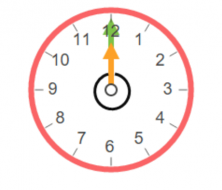Now, we’re going to see how we can calculate an angle that’s formed by the two hands of a clock at whatever time. We could use a protractor but, if we don’t have one handy, how can we calculate angles?

We can start by looking at the minute hand, the long hand that indicates the minutes.

The minute hand covers 360° when it completes its cycle, in other words, an hour. There are a total of 60 minutes in an hour, so each minute is 6°.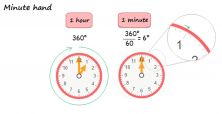Below, we’re going to see how the hour hand goes by, which is the short hand that indicates the hours.

If we divide a complete round (360°) by the total number of hours (12 hours), we get 30°. That is to say, the hour hand moves 30° per hour.

If in one hour, which is 60 minutes, the hour hand moves 30°, it’ll move 0.5° in one minute, as we can see in the next picture.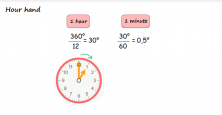Now, let’s get busy and calculate the angle formed by the hands that point to 5 o’clock sharp.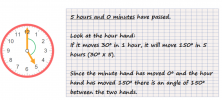What angle will the clock hands make after 10 minutes?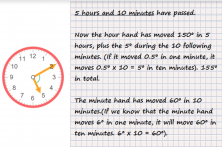If you want to practice more exercises on clocks and angles, sign on to Smartick and you’ll find plenty more!